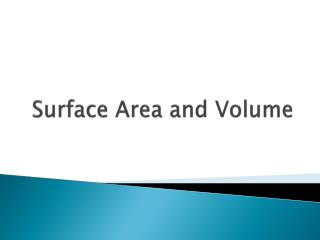DownloadDownload PresentationSurface Area and Volume

# Surface Area and Volume

Télécharger la présentation## Surface Area and Volume

- - - - - - - - - - - - - - - - - - - - - - - - - - - E N D - - - - - - - - - - - - - - - - - - - - - - - - - - -
##### Presentation Transcript

1. Surface Area and Volume

2. Learning Targets: • Meaning and calculation of area of non-rectangles • Meaning and calculation of surface area using nets • Meaning and calculation of volume • Angle relationships in figures • Pythagorean Theorem • Cross sections of 3D solids

3. Common Core 6th Grade: Area of triangles and special quadrilaterals; volume of rectangular prisms; surface area using nets 7th Grade: Area and circumference of circles; volume and SA of composite figures; scale drawings; cross-sections; angle relationships 8th Grade: Volume of cones, cylinders, spheres; transformations; Pythagorean Theorem; congruent and similar figures; angle relationships

4. NCTM Box Problem on Illuminations GSP simulation Simulation including graph

5. Basics – for intervention Table for 22: Real-World Geometry Problem

6. Area of rectangles

7. Area of rectangles

8. Area of parallelograms MSU Virtual Manipulatives for finding area

9. Area of triangles

10. Area of trapezoids

11. A little fun… Start with a 1x2 rectangle. Find the area. Then consider a 2x4 rectangle. How have the sides changed? How has the area changed? Find the area of a 3x6 rectangle.How have the sides changed?How has the area changed? Make a table to try a few more of these, following the same pattern. What might explain this pattern? What if you tried this with rectangular prisms and volume? s 2s

12. Don’t forget circles • GSP simulation of laying out slices of a circle to find its area

13. Nets and surface area Learning activities from Annenberg Online animations of unfolding cubes and rectangular prisms Find all 11 nets of a cubeon the handout

14. The diagram shows a prism constructed from two rectangular prisms. Draw the net for the solid and mark the lengths. Calculate the surface area of the solid.

15. Net of a pyramid Build one if you like. Will the other nets make a closed solid?

16. Net of a cylinder Unfolding a cylinder Huge Chrome Cylinder Box Unfolding

17. Finding Volume Sugar cubes and boxes at your table Annenberg Learner - Surface Area and Volume

18. 5.MD.3 Recognize volume as an attribute of solid figures and understand concepts of volume measurement. a. A cube with side length 1 unit, called a “unit cube,” is said to have “one cubic unit” of volume, and can be used to measure volume. b. A solid figure which can be packed without gaps or overlaps using n unit cubes is said to have a volume of n cubic units. 5.MD.4 Measure volumes by counting unit cubes, using cubic cm, cubic in, cubic ft, and improvised units.

19. 5.MD.5 Relate volume to the operations of multiplication and addition and solve real world and mathematical problems involving volume. a. Find the volume of a right rectangular prism with whole-number side lengths by packing it with unit cubes, and show that the volume is the same as would be found by multiplying the edge lengths, equivalently by multiplying the height by the area of the base. Represent three-fold whole-number products as volumes, e.g., to represent the associative property of multiplication. b. Apply the formulas V =(l)(w)(h) and V = (b)(h) for rectangular prisms to find volumes of right rectangular prisms with whole-number edge lengths in the context of solving real world and mathematical problems. c. Recognize volume as additive. Find volumes of solid figures composed of two non-overlapping right rectangular prisms by adding the volumes of the non-overlapping parts, applying this technique to solve real world problems.

20. Finding Volume Illuminations - Interactive Simulation of Volume

21. Toy Chest Generate possible dimensions for other toy chests that have a volume of 5400 square inches. Here is one: 12” high by 15” deep by 30” wide 9 x 15 x 30 = 4050

22. Each toy chest will be covered felt. Will the same amount of felt be needed for each chest regardless of the dimensions, since they all have the same volume?

23. Substituting values into formulas First, work through these problems. How much practice with 1a and 2a is needed? Would you give 1b/c and 2b as separate problems and not provide the formulas? How much support is needed to do 1b/c and 2b?

24. Recognize volume as additive The diagram shows a prism constructed from two rectangular prisms. Draw the net for the solid and mark the lengths. Calculate the surface area of the solid and its volume.

25. Cereal Box and Toy Chest • First, work through these problems. • Remembering that percent increase is in 7th grade, where would these problems fit in the curriculum?

26. Design a container • Where would this problem fit in the curriculum?

27. Volume of cylinders & pyramids

28. Cross-sections • Doing it with clay on YouTube

29. Angles Linear Pair Vertical Angles Exterior Angle of a Triangle 1 Exterior Angle of a Triangle 2

30. More Angles • Parallel lines cut by a transversal

31. Exterior angles of a triangle

32. Transformations • Two reflections equal a rotation • Explore a rotation

33. Pythagorean Theorem Do you have a proof you like? Proof by rearrangement Distance between two points in the plane AB2 + AC2 = CB2 4 + 36 = CB2 40 = CB2 6.32 = CB

34. Intervention approaches • Re-teaching the whole class • Based on weekly formative assessments • During individual or small group practice time

35. We would like your help